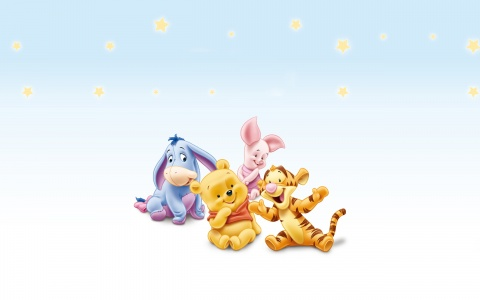WE USE COOKIES           ** FACEBOOK PAGE
This Disney wallpaper features Winnie the Pooh, Piglet, Tigger, and Eeyore as babies, as they are seen in the Disney baby brand. Based on the "Winnie the Pooh" works, by A.A. Milne and E.H. Shepard.## Download Disney Baby - Winnie the Pooh and Friends wallpaper | Leave a Comment

### function roundNumber(b,c){var a=Math.round(b*Math.pow(10,c))/Math.pow(10,c);return a}function resPrints(){var b=screen.width;var c=screen.height;var a=roundNumber(b/c,2);var d="Your display Resolution: <strong> "+b+" x "+c+" </strong> | Aspect Ratio:<strong> ";if(a==1.25||a==1.33){d+="Standard 4:3"}else{if(a==1.6||a==1.67){d+="Wide 8:5"}else{if(a==1.78){d+="HD 16:9"}else{if(a==1.76){d+="Mobile PSP"}else{if(a==0.75||(a==1.33&&b<=640)){d+="Mobile VGA"}else{if(a==0.6||(a==1.67&&b<=800)){d+="Mobile WVGA"}else{if(a==0.67||(a==1.5&&b==480)){d+="Mobile iPhone"}else{if(a==0.8){d+="Mobile Phone"}else{d="Unknown"}}}}}}}}d+="</strong>";document.write(d)}; resPrints();

Wallpaper details:
Added: Thursday, 19 April 2012 22:18
Downloads: 224592
Sub Categories:
License: Free for personal wallpaper use only